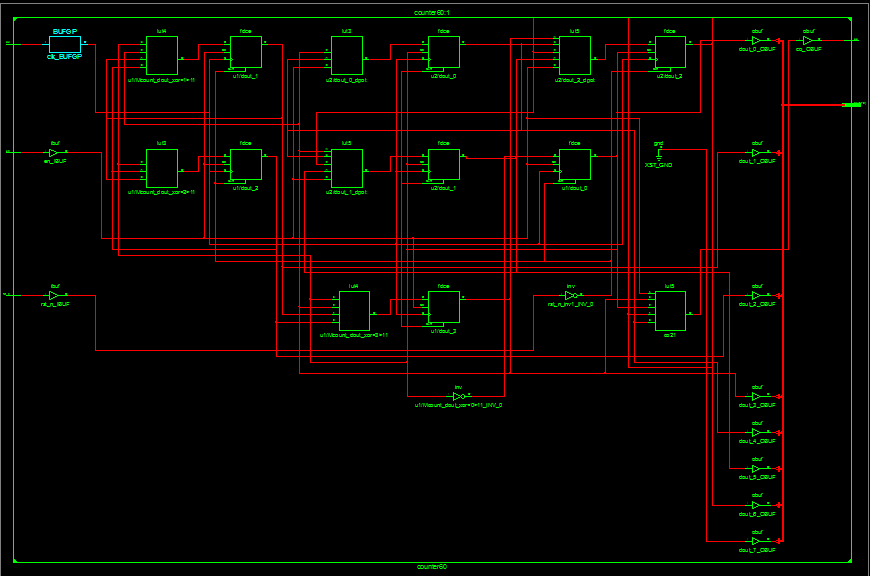级联模60计数器（Verilog HDL语言描述）（仿真与综合）

• 时间:

Verilog HDL语言描述

RTL Schematic

Technology Schematic

模60计数器

Verilog HDL语言描述

//模60计数器的Verilog HDL设计
module counter60(clk, rst_n, en, dout, co);

input clk, rst_n, en;
output[7:0] dout;
output co;
wire co10_1, co10, co6;
wire[3:0] dout10, dout6;

counter10 u1(.clk(clk), .rst_n(rst_n), .en(en), .dout(dout10), .co(co10_1)); //模10计数器的进位为co10_1
and u3(co10,en,co10_1); //co10_1与en的与为co10
counter6 u2(.clk(clk), .rst_n(rst_n), .en(co10), .dout(dout6), .co(co6)); //co10_1与en的与为co10,作为模6计数器的使能信号
and u4(co, co10, co6); //模6计数器的进位和模6的使能信号co10的与作为模60计数器的进位

assign dout = {dout6,dout10}; //模60计数器的输出，高位为模6计数器的输出，低位为模10计数器的输出，读法是8421BCD码读法

endmodule

//模6计数器模块
module counter6(clk, rst_n, en, dout, co);

input clk, rst_n, en;
output[3:0] dout;
reg [3:0] dout;
output co;

always@(posedge clk or negedge rst_n)
begin
if(!rst_n)
dout <= 4'b0000;        //系统复位，计数器清零
else if(en)
if(dout == 4'b0101)     //计数值达到5时，计数器清零
dout <= 4'b0000;
else
dout <= dout + 1'b1; //否则，计数器加1
else
dout <= dout;

end

assign co = dout&dout;  //当计数达到5(4'b1001)时，进位为1，计数值为其他，都没有进位

endmodule

//模10计数器模块
module counter10(clk, rst_n, en, dout, co);

input clk, rst_n, en;
output[3:0] dout;
reg [3:0] dout;
output co;

always@(posedge clk or negedge rst_n)
begin
if(!rst_n)
dout <= 4'b0000;        //系统复位，计数器清零
else if(en)
if(dout == 4'b1001)     //计数值达到5时，计数器清零
dout <= 4'b0000;
else
dout <= dout + 1'b1; //否则，计数器加1
else
dout <= dout;

end

assign co = dout&dout;  //当计数达到5(4'b1001)时，进位为1，计数值为其他，都没有进位

endmodule

测试文件

//模10计数器的测试文件
`timescale 1ns/1ps
module counter60_tb;

reg clk, rst_n, en;
wire[7:0] dout;
wire co;

//时钟设计周期为2ns
always
begin
#1 clk = ~clk;
end

//初始化
initial
begin
clk = 1'b0;
rst_n = 1'b1;
en = 1'b0;
#2 rst_n = 1'b0;
#2 rst_n = 1'b1; en = 1'b1;    //计数使能信号有效，且不复位

end

counter60 u5(.clk(clk), .rst_n(rst_n), .en(en), .dout(dout), .co(co));

endmodule

仿真波形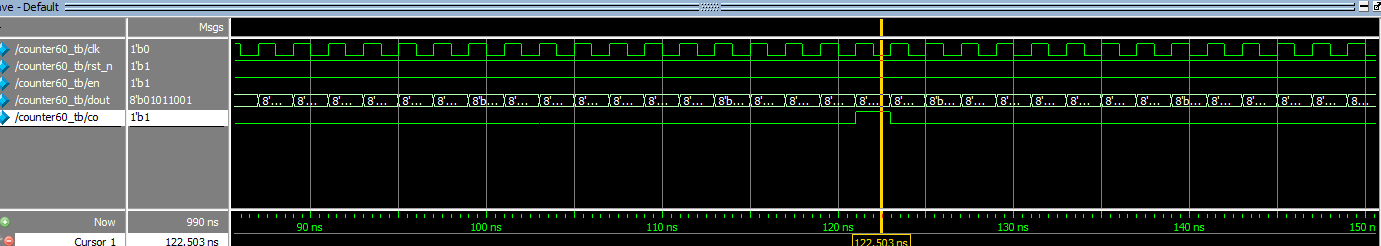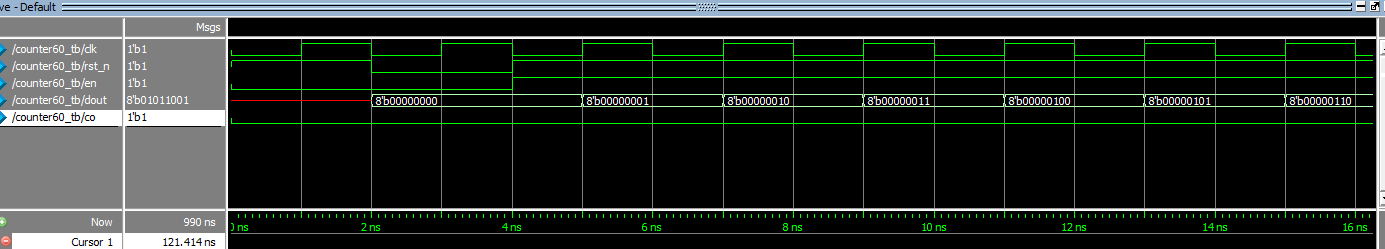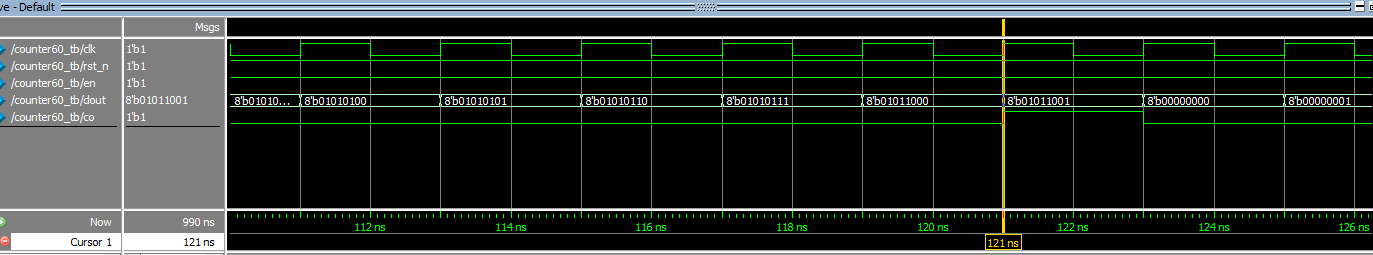RTL Schematic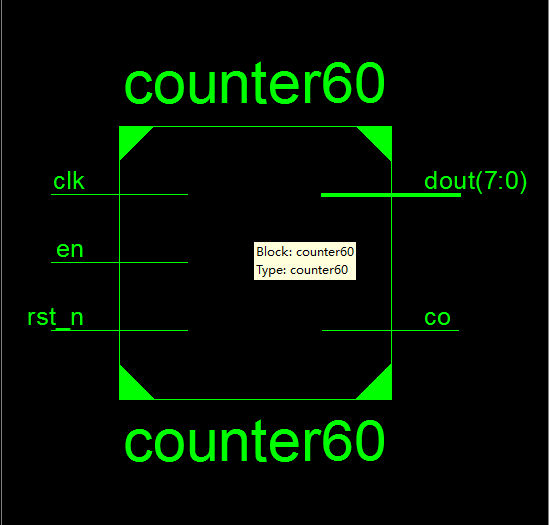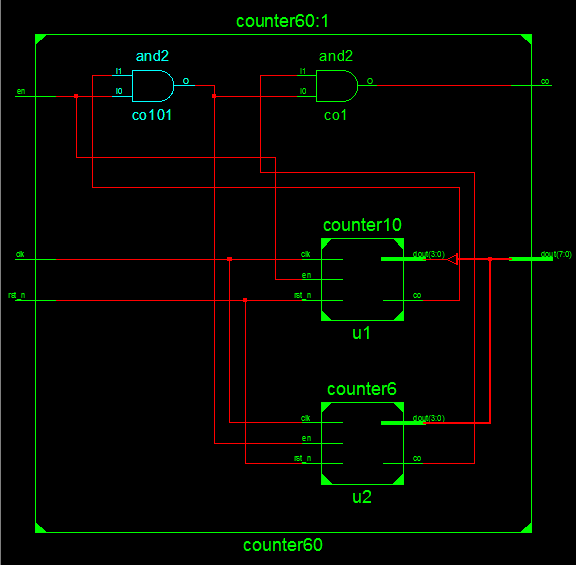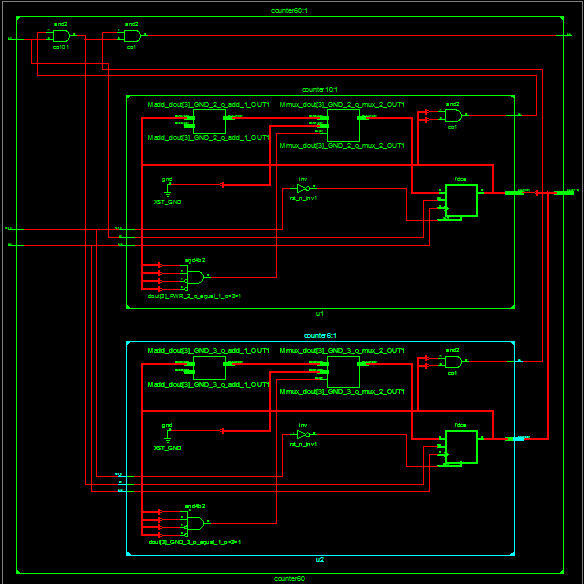Technology Schematic